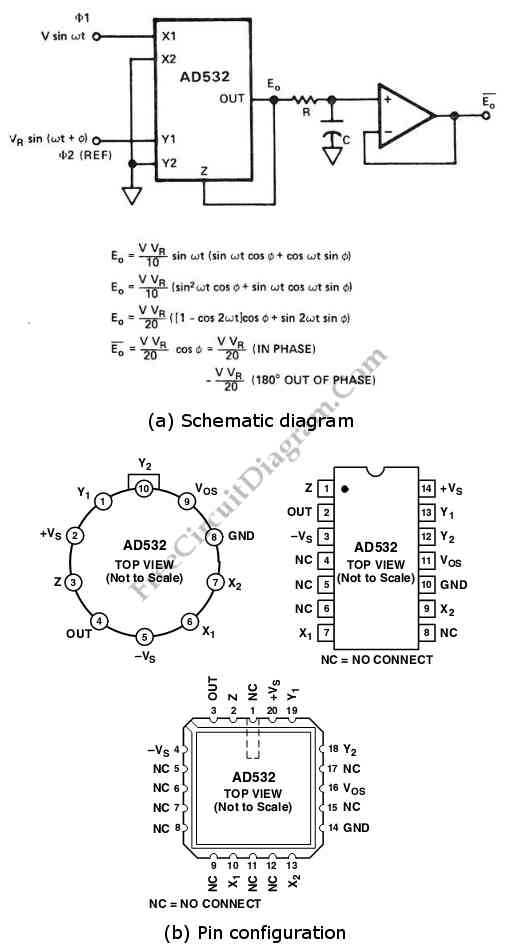## Analog Multiplier-Divider with 4136 Op-Amps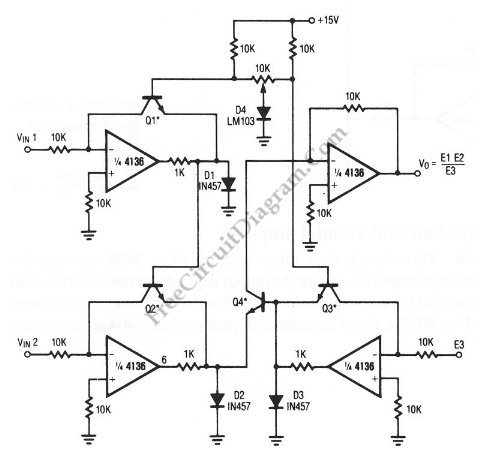This unique circuit below can be used as divider and multiplier. This circuit is formed by all four sections of a 4136 . The Q1 through Q4 can be any PNP transistor, but that must matched transistors. The transistor matching determine the acurracy of the divider and multiplier circuit. Here is the schematic diagram of the circuit:

## 30-Khz Bandwidth High Linearity Opto-Isolated Amplifier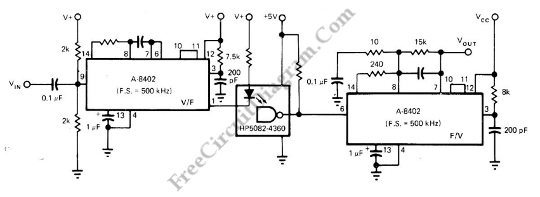Using optocoupler to directly transfer a linear (analog) voltage would produce  a large unpredictable linearity error, which is also sensitive to temperature changes. By using frequency modulation, the total system’s linearity will  depend only on the linearity of the V/F and F/V converter, which is fortunately cancel each other if we use similar devices with similar non-linearity error characteristic. A […]

## Sound-Activated Lamp (Relay/Switch)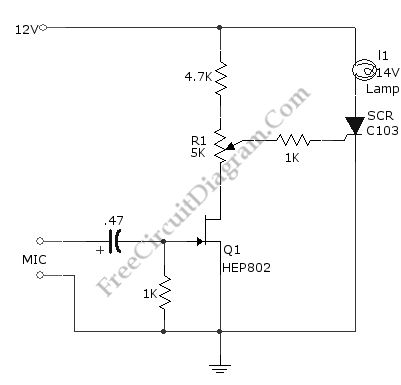This simple circuit shown int the schematic diagram actives the switch using sound. We can use this circuit for various applications, such as automatic (sound-controlled) disco light or car’s LED light show.  The Q1 amplify the audio from mic. The R1 is used to adjust the peak of signal to greater than about 0.7 volts, act as sensitivity adjuster. A […]

## Selectable Voltages 6V, 9V, and 12V Linear Voltage Regulator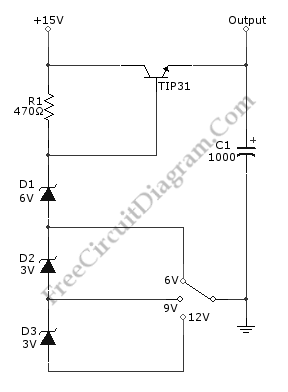We can build a multiple voltage power supply 6, 9, and 12V  (AC-DC Adapter) with the circuit shown in the following schematic diagram.  Not only provide multiple voltage output with single voltage supply, this circuit add the benefit of regulating the voltage for better stability. The TIP31 transistor should be installed with proper heat-sink to prevent overheating. A transformer […]

## Digital Encoder Circuit Using Stepper Motor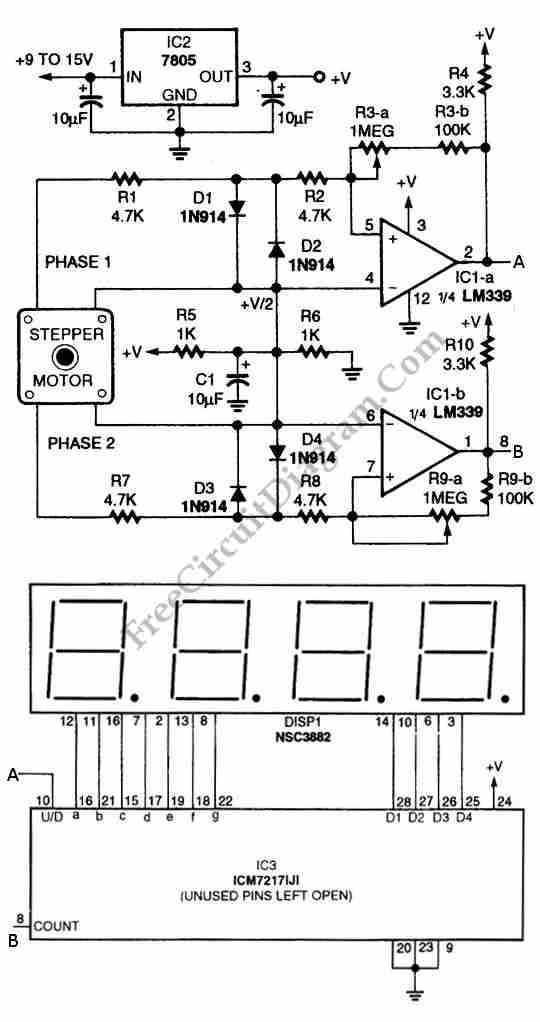Using circuit depicted in the schematic diagram below, the direction and shaft rotation of stepper motor can be seen on the LED display. Alternative to digital rotation encoder as a digital encoder input, this circuit uses a stepper motor. Here is the schematic diagram of the circuit:

## Wideband Buffer using FET and Bipolar Transistor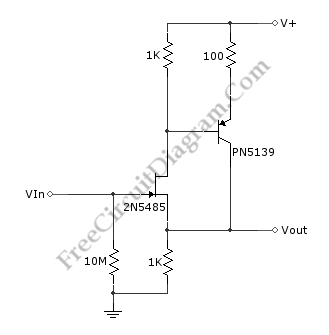The schematic diagram shown below is a wide-band buffer circuit. This circuit is formed by FET and transistor. This circuit consist of series-feedback buffer which act as wideband unity-gain amplifier. This wideband unity-gain amplifier has high input impedance due to the low input impedance of 2N5485 FET. Here is the schematic diagram of the circuit: